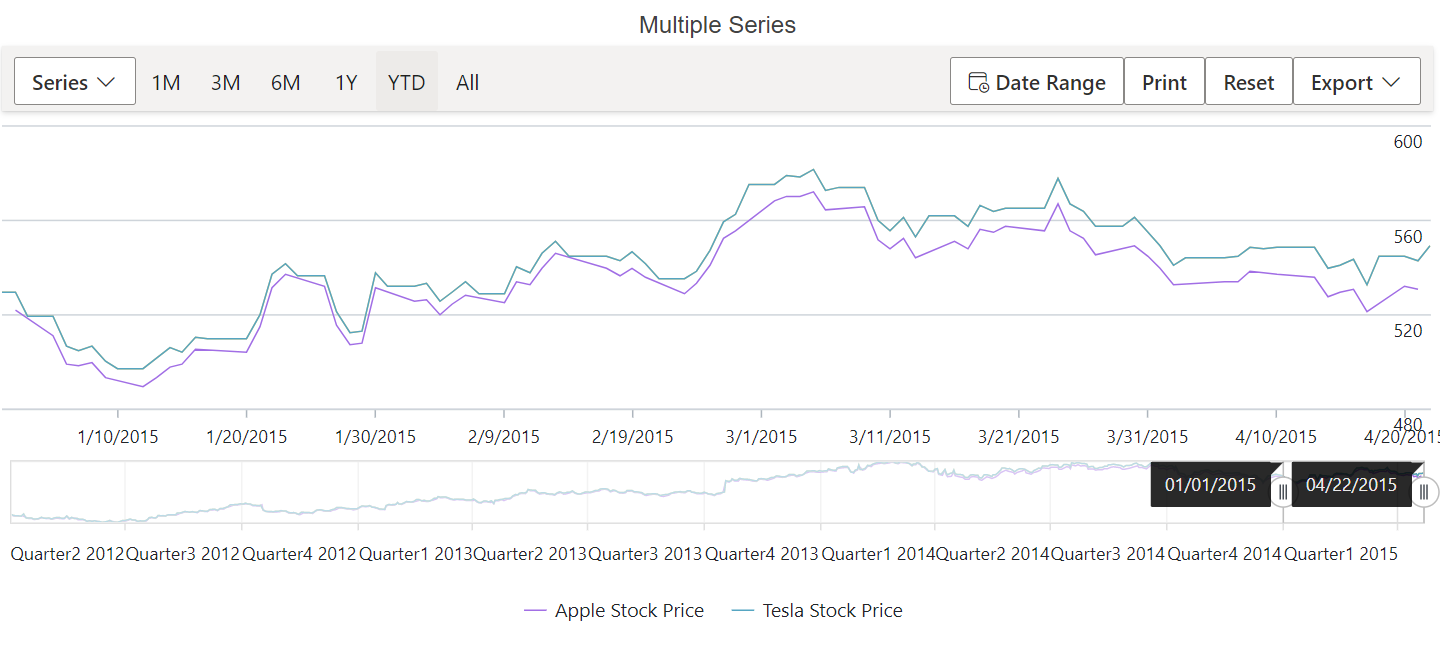# Legend in Blazor Stock Chart Component

30 Nov 202224 minutes to read

Legend provides information about the series rendered in the Stock Chart. Legend can be added to a Stock Chart by enabling the Visible option in the StockChartLegendSettings.

## Enable Legend

To display the legend for the Stock Chart, set the Visible property in StockChartLegendSettings to true.

``````@using Syncfusion.Blazor.Charts

<SfStockChart Title="AAPL Stock Price">
<StockChartLegendSettings Visible="true"></StockChartLegendSettings>
<StockChartSeriesCollection>
<StockChartSeries DataSource="@StockDetails" XName="Date" High="High" Low="Low" Open="Open" Close="Close" Name="Apple  Inc" Type="ChartSeriesType.Candle"></StockChartSeries>
</StockChartSeriesCollection>
</SfStockChart>

@code {
public class ChartData
{
public DateTime Date { get; set; }
public Double Open { get; set; }
public Double Low { get; set; }
public Double Close { get; set; }
public Double High { get; set; }
public Double Volume { get; set; }
}

public List<ChartData> StockDetails = new List<ChartData>
{
new ChartData { Date = new DateTime(2012, 04, 02), Open= 85.9757, High = 90.6657,Low = 85.7685, Close = 90.5257,Volume = 660187068},
new ChartData { Date = new DateTime(2012, 04, 09), Open= 89.4471, High = 92,Low = 86.2157, Close = 86.4614,Volume = 912634864},
new ChartData { Date = new DateTime(2012, 04, 16), Open= 87.1514, High = 88.6071,Low = 81.4885, Close = 81.8543,Volume = 1221746066},
new ChartData { Date = new DateTime(2012, 04, 23), Open= 81.5157, High = 88.2857,Low = 79.2857, Close = 86.1428,Volume = 965935749},
new ChartData { Date = new DateTime(2012, 04, 30), Open= 85.4, High =  85.4857,Low = 80.7385, Close = 80.75,Volume = 615249365},
};
}``````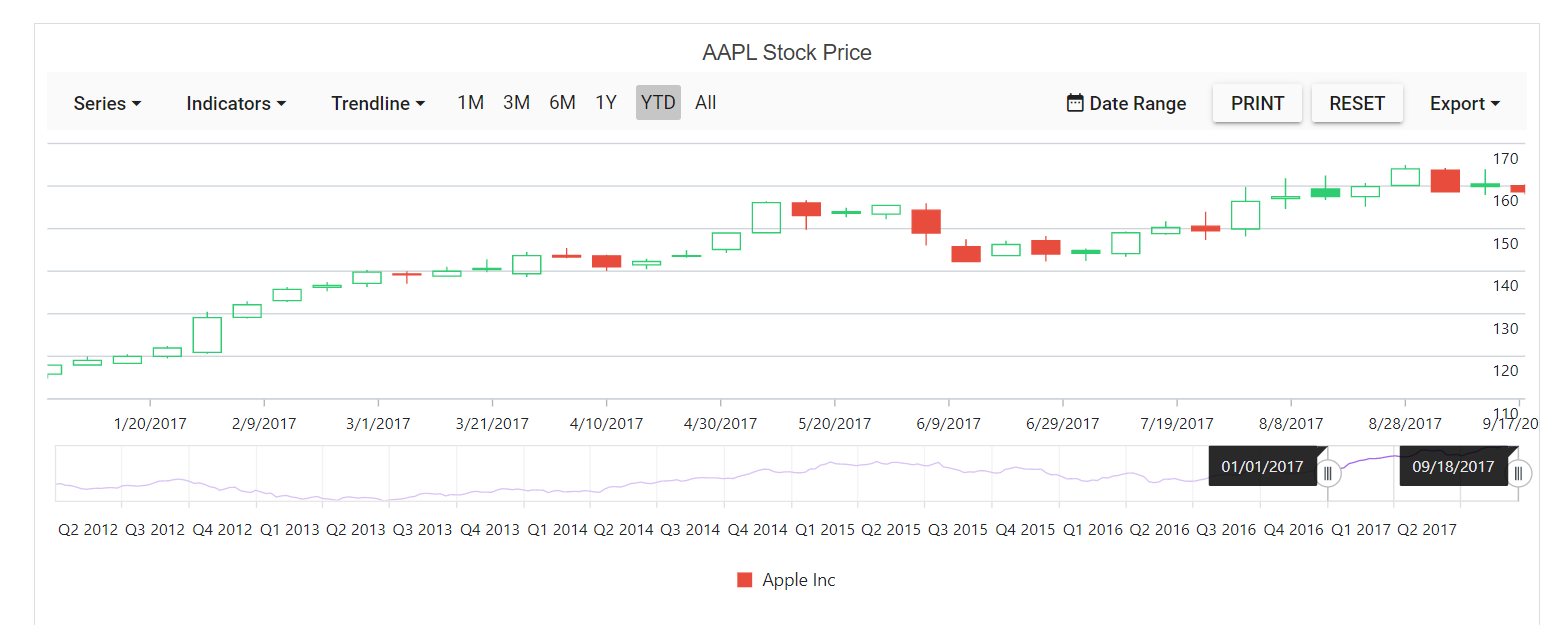## Legend Position

By using the Position property, legend can be placed at Left, Right, Top, Bottom or Custom of the Stock Chart. The legend is positioned at the bottom of the Stock Chart, by default.

``````@using Syncfusion.Blazor.Charts

<SfStockChart Title="AAPL Stock Price">
<StockChartLegendSettings Visible="true" Position="LegendPosition.Top"></StockChartLegendSettings>
<StockChartSeriesCollection>
<StockChartSeries DataSource="@StockDetails" XName="Date" High="High" Low="Low" Open="Open" Close="Close" Name="Apple Inc" Type="ChartSeriesType.Candle"></StockChartSeries>
</StockChartSeriesCollection>
</SfStockChart>

@code {
public class ChartData
{
public DateTime Date { get; set; }
public Double Open { get; set; }
public Double Low { get; set; }
public Double Close { get; set; }
public Double High { get; set; }
public Double Volume { get; set; }
}

public List<ChartData> StockDetails = new List<ChartData>
{
new ChartData { Date = new DateTime(2012, 04, 02), Open= 85.9757, High = 90.6657,Low = 85.7685, Close = 90.5257,Volume = 660187068},
new ChartData { Date = new DateTime(2012, 04, 09), Open= 89.4471, High = 92,Low = 86.2157, Close = 86.4614,Volume = 912634864},
new ChartData { Date = new DateTime(2012, 04, 16), Open= 87.1514, High = 88.6071,Low = 81.4885, Close = 81.8543,Volume = 1221746066},
new ChartData { Date = new DateTime(2012, 04, 23), Open= 81.5157, High = 88.2857,Low = 79.2857, Close = 86.1428,Volume = 965935749},
new ChartData { Date = new DateTime(2012, 04, 30), Open= 85.4, High =  85.4857,Low = 80.7385, Close = 80.75,Volume = 615249365},
};
}``````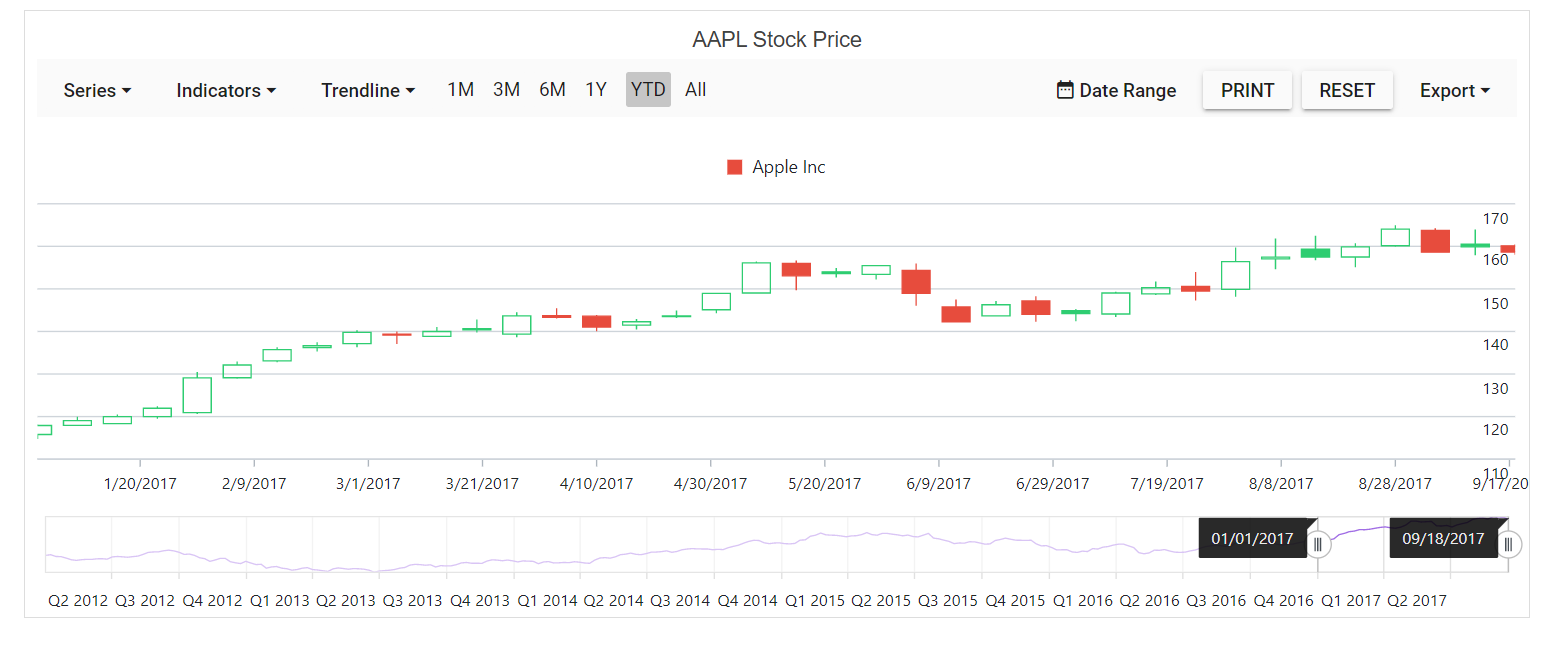The Custom position helps to position the legend anywhere in the Stock Chart using x and y coordinates.

``````@using Syncfusion.Blazor.Charts

<SfStockChart Title="AAPL Stock Price">
<StockChartLegendSettings Visible="true" Position="LegendPosition.Custom">
<StockChartLocation X="50" Y="50"></StockChartLocation>
<StockChartLegendBorder Color="Black" Width="2"></StockChartLegendBorder>
</StockChartLegendSettings>
<StockChartSeriesCollection>
<StockChartSeries DataSource="@StockDetails" XName="Date" High="High" Low="Low" Open="Open" Close="Close" Name="Apple Inc" Type="ChartSeriesType.Candle"></StockChartSeries>
</StockChartSeriesCollection>
</SfStockChart>

@code {
public class ChartData
{
public DateTime Date { get; set; }
public Double Open { get; set; }
public Double Low { get; set; }
public Double Close { get; set; }
public Double High { get; set; }
public Double Volume { get; set; }
}

public List<ChartData> StockDetails = new List<ChartData>
{
new ChartData { Date = new DateTime(2012, 04, 02), Open= 85.9757, High = 90.6657,Low = 85.7685, Close = 90.5257,Volume = 660187068},
new ChartData { Date = new DateTime(2012, 04, 09), Open= 89.4471, High = 92,Low = 86.2157, Close = 86.4614,Volume = 912634864},
new ChartData { Date = new DateTime(2012, 04, 16), Open= 87.1514, High = 88.6071,Low = 81.4885, Close = 81.8543,Volume = 1221746066},
new ChartData { Date = new DateTime(2012, 04, 23), Open= 81.5157, High = 88.2857,Low = 79.2857, Close = 86.1428,Volume = 965935749},
new ChartData { Date = new DateTime(2012, 04, 30), Open= 85.4, High =  85.4857,Low = 80.7385, Close = 80.75,Volume = 615249365},
};
}``````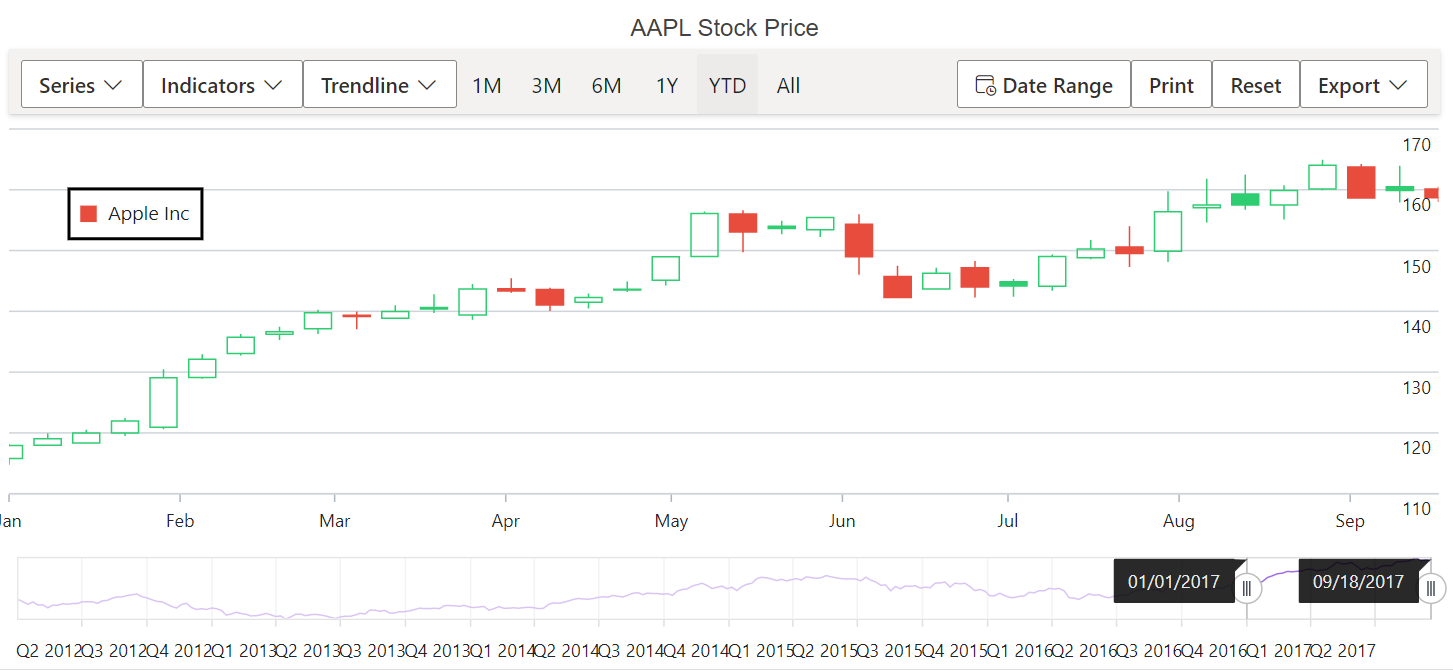## Reverse Legend

You can reverse the order of the legend items by using the Reversed property. By default, legend for the first series in the collection will be placed first.

``````@using Syncfusion.Blazor.Charts

<SfStockChart Title="AAPL Stock Price">
<StockChartLegendSettings Visible="true" Reversed="true">
</StockChartLegendSettings>
<StockChartSeriesCollection>

<StockChartSeries DataSource="@StockDetails" Type="ChartSeriesType.Line" XName="Date" High="High" Low="Low" Open="Open" Close="Close" Name="Apple Stock Price"></StockChartSeries>

<StockChartSeries DataSource="@StockDetails" Type="ChartSeriesType.Line" XName="Date" High="High" Low="Low" Open="Open" Close="Close" Name="Google Stock Price"></StockChartSeries>

<StockChartSeries DataSource="@StockDetails" Type="ChartSeriesType.Spline"XName="Date" High="High" Low="Low" Open="Open" Close="Close" Name="Tesla Stock Price"></StockChartSeries>

</StockChartSeriesCollection>
</SfStockChart>

@code {
public class ChartData
{
public DateTime Date { get; set; }
public Double Open { get; set; }
public Double Low { get; set; }
public Double Close { get; set; }
public Double High { get; set; }
public Double Volume { get; set; }
}

public List<ChartData> StockDetails = new List<ChartData>
{
new ChartData { Date = new DateTime(2012, 04, 02), Open= 85.9757, High = 90.6657,Low = 85.7685, Close = 90.5257,Volume = 660187068},
new ChartData { Date = new DateTime(2012, 04, 09), Open= 89.4471, High = 92,Low = 86.2157, Close = 86.4614,Volume = 912634864},
new ChartData { Date = new DateTime(2012, 04, 16), Open= 87.1514, High = 88.6071,Low = 81.4885, Close = 81.8543,Volume = 1221746066},
new ChartData { Date = new DateTime(2012, 04, 23), Open= 81.5157, High = 88.2857,Low = 79.2857, Close = 86.1428,Volume = 965935749},
new ChartData { Date = new DateTime(2012, 04, 30), Open= 85.4, High =  85.4857,Low = 80.7385, Close = 80.75,Volume = 615249365},
};
}``````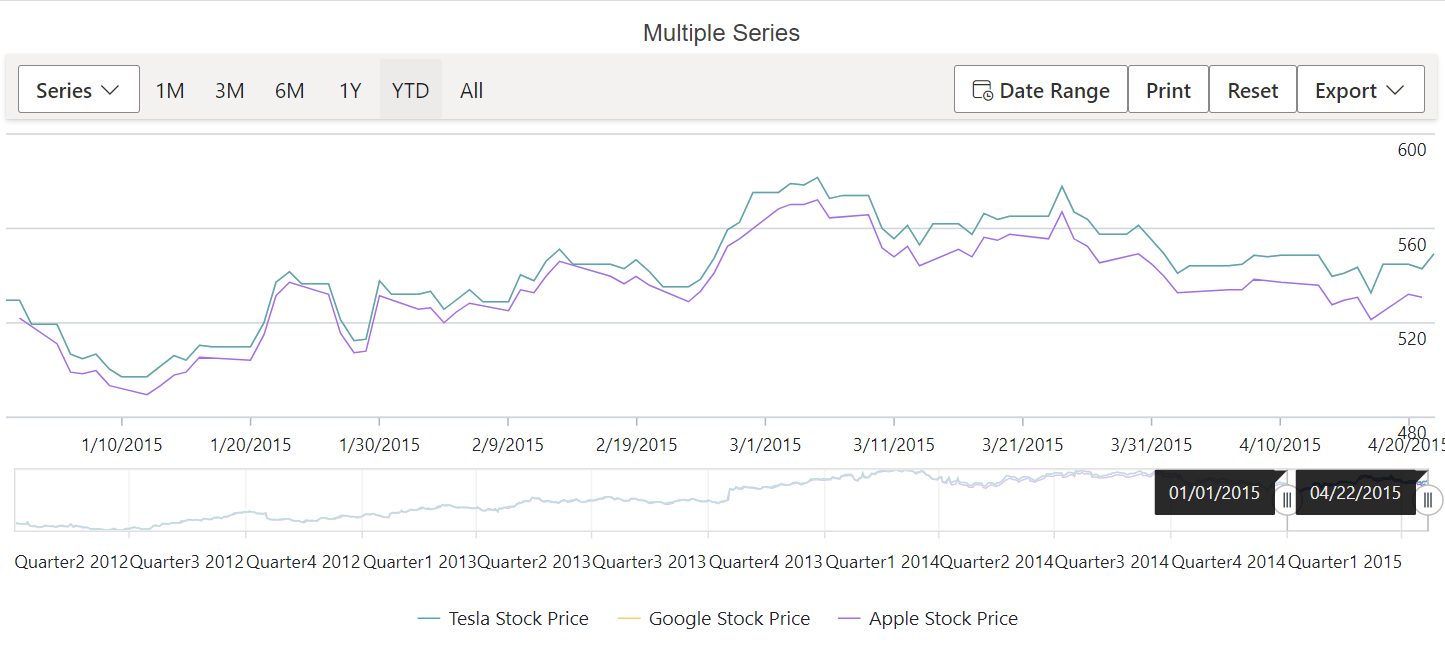## Legend Alignment

Using the Alignment property, place the legend in Centre, Far, or Near alignment.

``````@using Syncfusion.Blazor.Charts

<SfStockChart Title="AAPL Stock Price">
<StockChartLegendSettings Visible="true" Alignment="Alignment.Near">
<StockChartLegendBorder Color="Black" Width="2"></StockChartLegendBorder>
</StockChartLegendSettings>
<StockChartSeriesCollection>
<StockChartSeries DataSource="@StockDetails"  XName="Date" High="High" Low="Low" Open="Open" Close="Close" Name="Apple Inc" Type="ChartSeriesType.Candle"></StockChartSeries>
</StockChartSeriesCollection>
</SfStockChart>

@code {
public class ChartData
{
public DateTime Date { get; set; }
public Double Open { get; set; }
public Double Low { get; set; }
public Double Close { get; set; }
public Double High { get; set; }
public Double Volume { get; set; }
}

public List<ChartData> StockDetails = new List<ChartData>
{
new ChartData { Date = new DateTime(2012, 04, 02), Open= 85.9757, High = 90.6657,Low = 85.7685, Close = 90.5257,Volume = 660187068},
new ChartData { Date = new DateTime(2012, 04, 09), Open= 89.4471, High = 92,Low = 86.2157, Close = 86.4614,Volume = 912634864},
new ChartData { Date = new DateTime(2012, 04, 16), Open= 87.1514, High = 88.6071,Low = 81.4885, Close = 81.8543,Volume = 1221746066},
new ChartData { Date = new DateTime(2012, 04, 23), Open= 81.5157, High = 88.2857,Low = 79.2857, Close = 86.1428,Volume = 965935749},
new ChartData { Date = new DateTime(2012, 04, 30), Open= 85.4, High =  85.4857,Low = 80.7385, Close = 80.75,Volume = 615249365},
};
}``````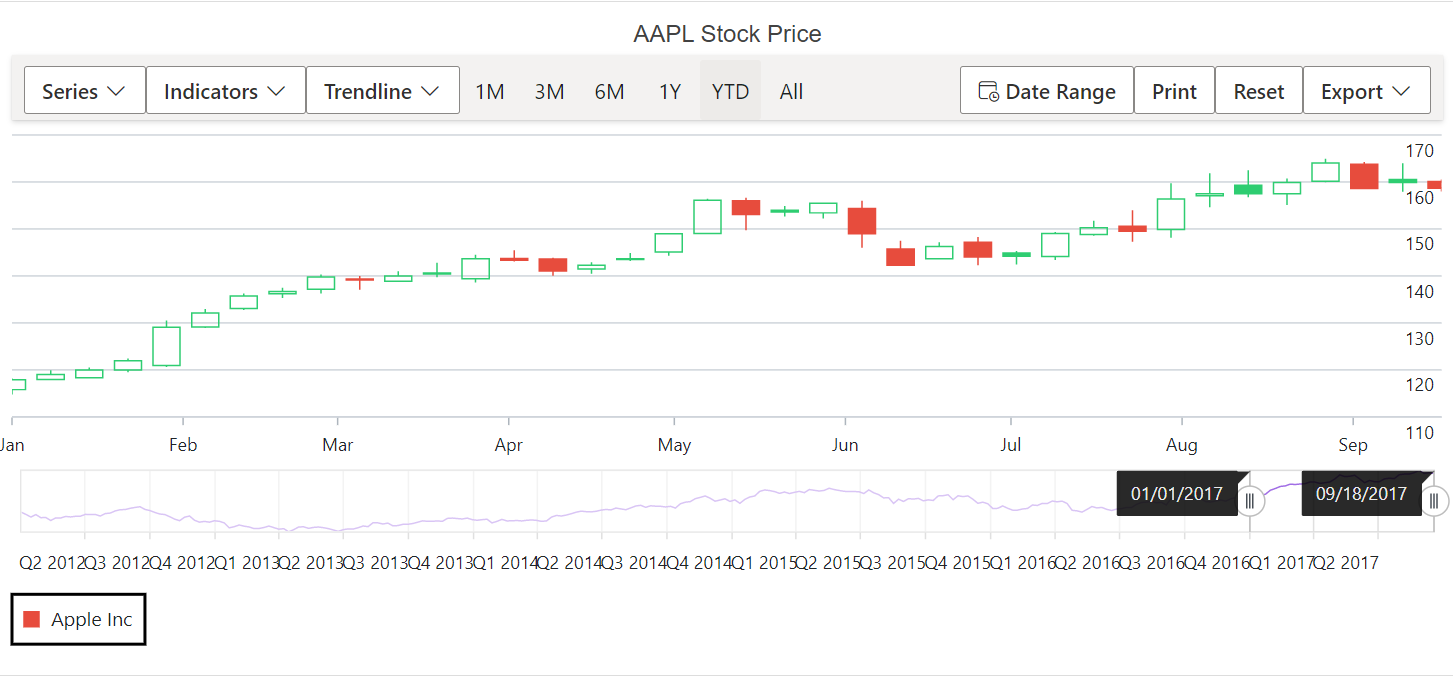## Legend Customization

### Legend Shape

The LegendShape property in the Series can be used to change the shape of the legend icon. The default icon shape for legends is SeriesType.

``````@using Syncfusion.Blazor.Charts

<SfStockChart Title="AAPL Stock Price">
<StockChartLegendSettings Visible="true" ShapeHeight="13" ShapeWidth="13">
</StockChartLegendSettings>
<StockChartSeriesCollection>
<StockChartSeries DataSource="@StockDetails" XName="Date" High="High" Low="Low" Open="Open" Close="Close" Name="Apple Inc" Type="ChartSeriesType.Candle"  LegendShape="LegendShape.Diamond"></StockChartSeries>
</StockChartSeriesCollection>
</SfStockChart>

@code {
public class ChartData
{
public DateTime Date { get; set; }
public Double Open { get; set; }
public Double Low { get; set; }
public Double Close { get; set; }
public Double High { get; set; }
public Double Volume { get; set; }
}

public List<ChartData> StockDetails = new List<ChartData>
{
new ChartData { Date = new DateTime(2012, 04, 02), Open= 85.9757, High = 90.6657,Low = 85.7685, Close = 90.5257,Volume = 660187068},
new ChartData { Date = new DateTime(2012, 04, 09), Open= 89.4471, High = 92,Low = 86.2157, Close = 86.4614,Volume = 912634864},
new ChartData { Date = new DateTime(2012, 04, 16), Open= 87.1514, High = 88.6071,Low = 81.4885, Close = 81.8543,Volume = 1221746066},
new ChartData { Date = new DateTime(2012, 04, 23), Open= 81.5157, High = 88.2857,Low = 79.2857, Close = 86.1428,Volume = 965935749},
new ChartData { Date = new DateTime(2012, 04, 30), Open= 85.4, High =  85.4857,Low = 80.7385, Close = 80.75,Volume = 615249365},
};
}``````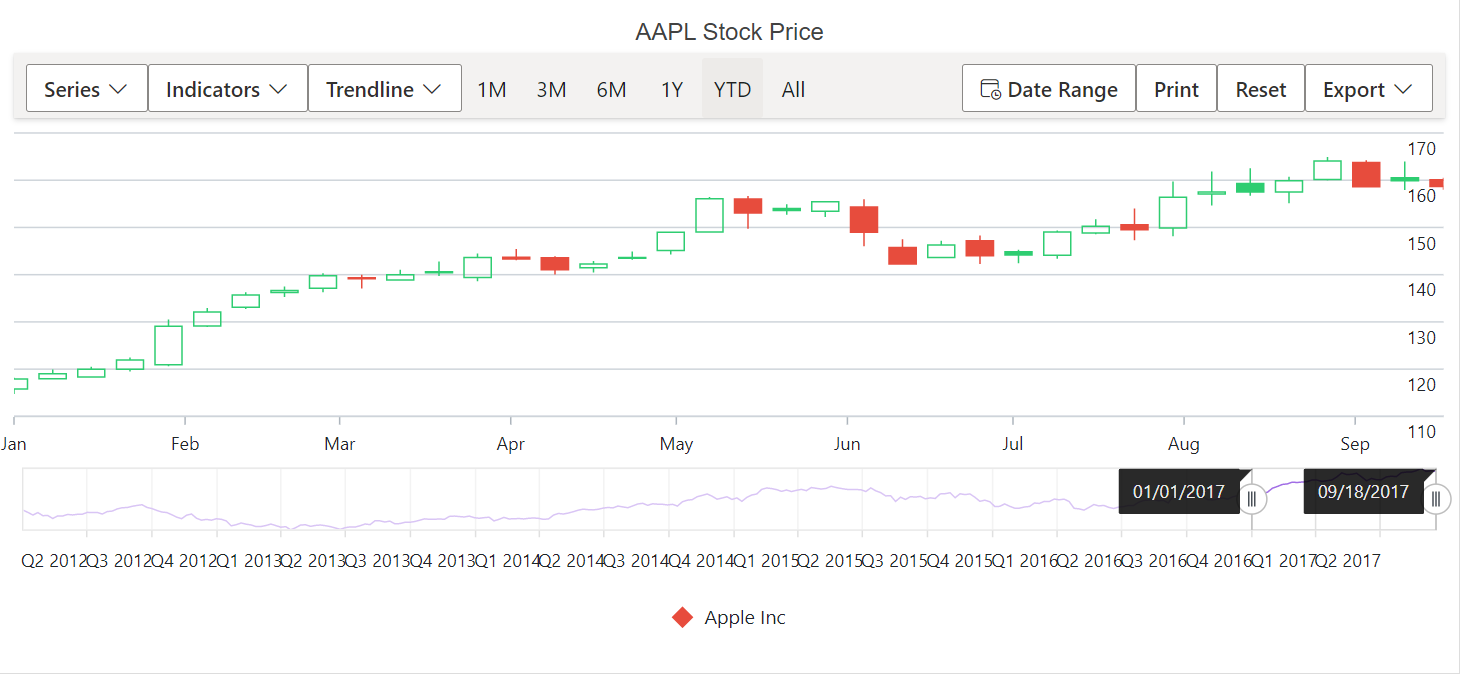### Legend Size

When the legend is placed on the top or bottom of the Stock Chart, it takes up 20% - 25% of the Stock Chart’s height, and 20% - 25% of the Stock Chart’s width when it is positioned on the left or right side of the Stock Chart. So, the Width and Height properties can be used to adjust the default legend size.

``````@using Syncfusion.Blazor.Charts

<SfStockChart Title="AAPL Stock Price">
<StockChartLegendSettings Visible="true" Height="50" Width="300">
<StockChartLegendBorder Color="blue" Width="1"></StockChartLegendBorder>
</StockChartLegendSettings>
<StockChartSeriesCollection>
<StockChartSeries DataSource="@StockDetails" Type="ChartSeriesType.Line" XName="Date" High="High" Low="Low" Open="Open" Close="Close" Name="Apple Stock Price"></StockChartSeries>
<StockChartSeries DataSource="@StockDetails" Type="ChartSeriesType.Line" XName="Date" High="High" Low="Low" Open="Open" Close="Close" Name="Google Stock Price"></StockChartSeries>
</StockChartSeriesCollection>
</SfStockChart>

@code {
public class ChartData
{
public DateTime Date { get; set; }
public Double Open { get; set; }
public Double Low { get; set; }
public Double Close { get; set; }
public Double High { get; set; }
public Double Volume { get; set; }
}

public List<ChartData> StockDetails = new List<ChartData>
{
new ChartData { Date = new DateTime(2012, 04, 02), Open= 85.9757, High = 90.6657,Low = 85.7685, Close = 90.5257,Volume = 660187068},
new ChartData { Date = new DateTime(2012, 04, 09), Open= 89.4471, High = 92,Low = 86.2157, Close = 86.4614,Volume = 912634864},
new ChartData { Date = new DateTime(2012, 04, 16), Open= 87.1514, High = 88.6071,Low = 81.4885, Close = 81.8543,Volume = 1221746066},
new ChartData { Date = new DateTime(2012, 04, 23), Open= 81.5157, High = 88.2857,Low = 79.2857, Close = 86.1428,Volume = 965935749},
new ChartData { Date = new DateTime(2012, 04, 30), Open= 85.4, High =  85.4857,Low = 80.7385, Close = 80.75,Volume = 615249365},
};
}``````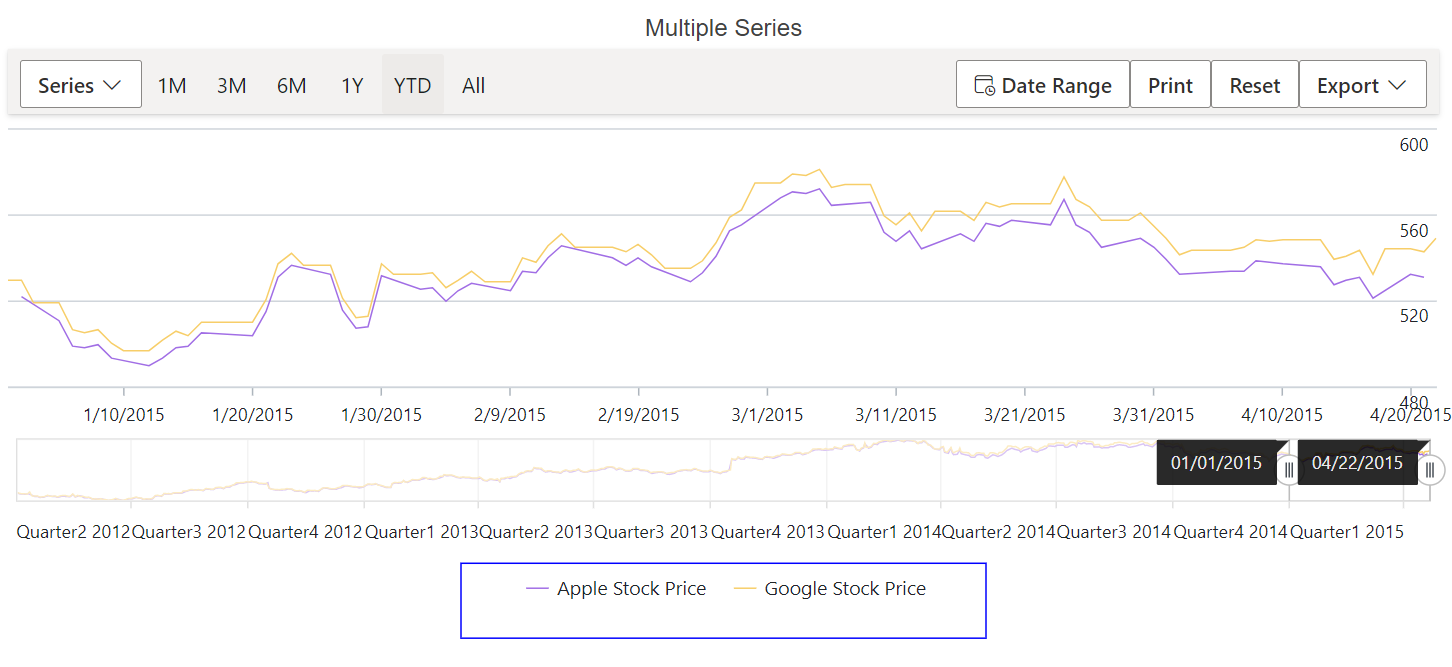### Legend Shape Size

The ShapeHeight and ShapeWidth properties can be used to adjust the dimensions of the legend shape.

``````@using Syncfusion.Blazor.Charts

<SfStockChart Title="AAPL Stock Price">
<StockChartLegendSettings Visible="true" Height="50" Width="300" ShapeHeight="15" ShapeWidth="15">
</StockChartLegendSettings>
<StockChartSeriesCollection>
<StockChartSeries DataSource="@StockDetails" XName="Date" High="High" Low="Low" Open="Open" Close="Close" Name="Apple Inc" Type="ChartSeriesType.Candle"></StockChartSeries>
</StockChartSeriesCollection>
</SfStockChart>

@code {
public class ChartData
{
public DateTime Date { get; set; }
public Double Open { get; set; }
public Double Low { get; set; }
public Double Close { get; set; }
public Double High { get; set; }
public Double Volume { get; set; }
}

public List<ChartData> StockDetails = new List<ChartData>
{
new ChartData { Date = new DateTime(2012, 04, 02), Open= 85.9757, High = 90.6657,Low = 85.7685, Close = 90.5257,Volume = 660187068},
new ChartData { Date = new DateTime(2012, 04, 09), Open= 89.4471, High = 92,Low = 86.2157, Close = 86.4614,Volume = 912634864},
new ChartData { Date = new DateTime(2012, 04, 16), Open= 87.1514, High = 88.6071,Low = 81.4885, Close = 81.8543,Volume = 1221746066},
new ChartData { Date = new DateTime(2012, 04, 23), Open= 81.5157, High = 88.2857,Low = 79.2857, Close = 86.1428,Volume = 965935749},
new ChartData { Date = new DateTime(2012, 04, 30), Open= 85.4, High =  85.4857,Low = 80.7385, Close = 80.75,Volume = 615249365},
};
}``````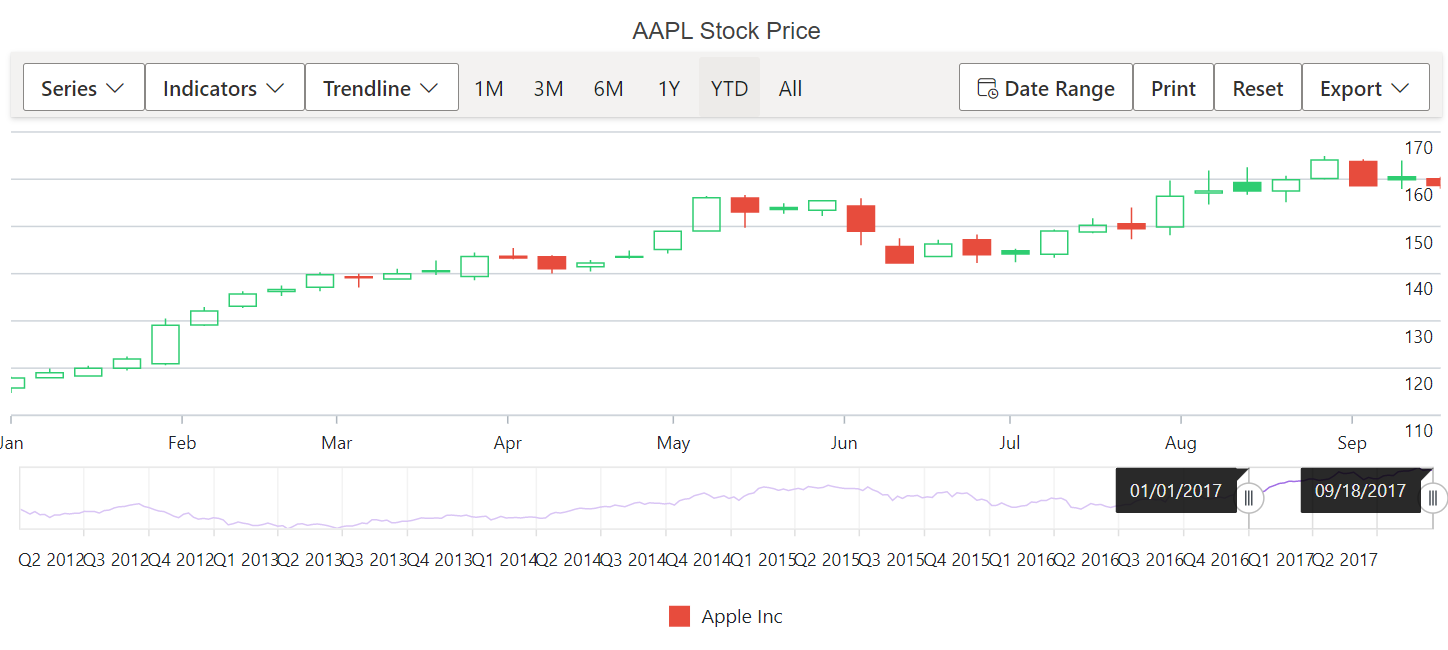The ItemPadding property can be used to adjust the space between the legend items.

``````@using Syncfusion.Blazor.Charts

<SfStockChart Title="AAPL Stock Price">
</StockChartLegendSettings>
<StockChartSeriesCollection>
<StockChartSeries DataSource="@StockDetails" Type="ChartSeriesType.Line" XName="Date" High="High" Low="Low" Open="Open" Close="Close" Name="Apple"></StockChartSeries>
<StockChartSeries DataSource="@StockDetails" Type="ChartSeriesType.Line" XName="Date" High="High" Low="Low" Open="Open" Close="Close" Name="Google"></StockChartSeries>
</StockChartSeriesCollection>
</SfStockChart>

@code {
public class ChartData
{
public DateTime Date { get; set; }
public Double Open { get; set; }
public Double Low { get; set; }
public Double Close { get; set; }
public Double High { get; set; }
public Double Volume { get; set; }
}

public List<ChartData> StockDetails = new List<ChartData>
{
new ChartData { Date = new DateTime(2012, 04, 02), Open= 85.9757, High = 90.6657,Low = 85.7685, Close = 90.5257,Volume = 660187068},
new ChartData { Date = new DateTime(2012, 04, 09), Open= 89.4471, High = 92,Low = 86.2157, Close = 86.4614,Volume = 912634864},
new ChartData { Date = new DateTime(2012, 04, 16), Open= 87.1514, High = 88.6071,Low = 81.4885, Close = 81.8543,Volume = 1221746066},
new ChartData { Date = new DateTime(2012, 04, 23), Open= 81.5157, High = 88.2857,Low = 79.2857, Close = 86.1428,Volume = 965935749},
new ChartData { Date = new DateTime(2012, 04, 30), Open= 85.4, High =  85.4857,Low = 80.7385, Close = 80.75,Volume = 615249365},
};
}``````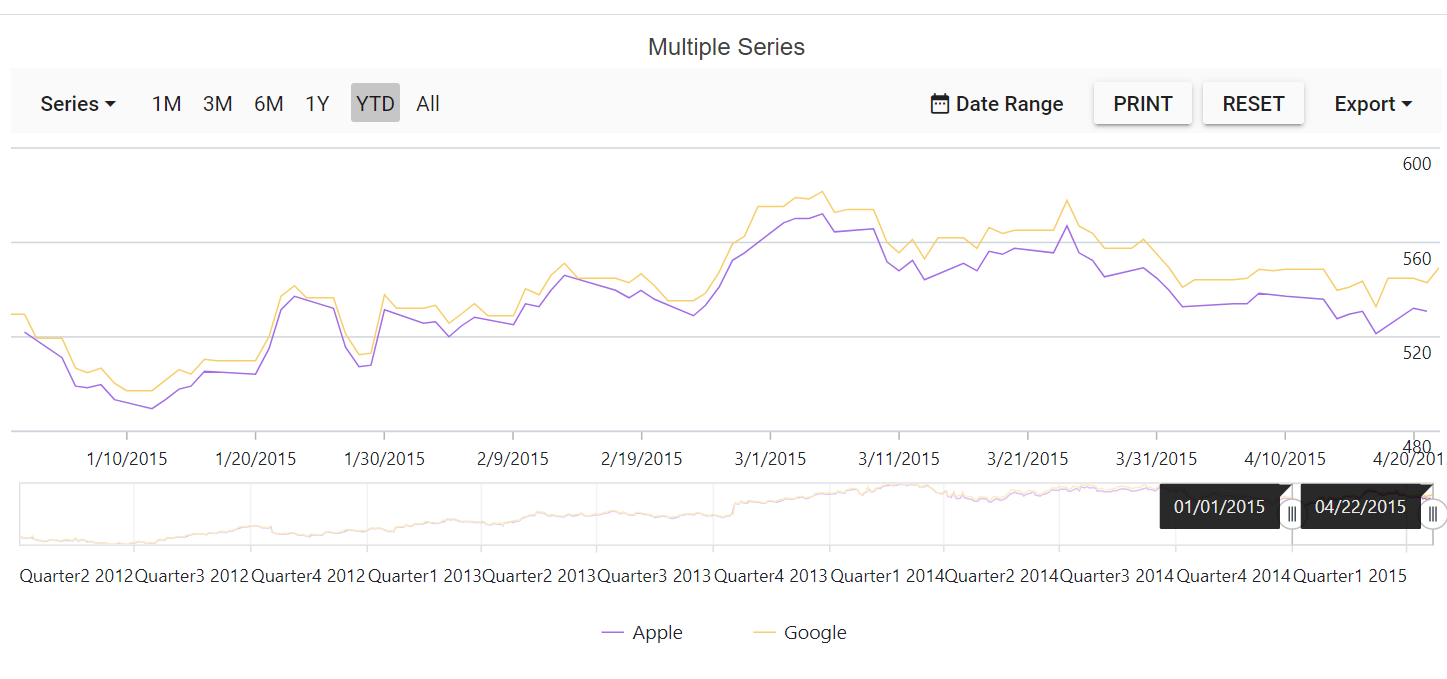### Legend Paging

When the legend items exceed legend bounds, paging will be enabled by default. End user can view each legend item using the navigation buttons to navigate between pages.

``````@using Syncfusion.Blazor.Charts

<SfStockChart Title="AAPL Stock Price">
</StockChartLegendSettings>
<StockChartSeriesCollection>
<StockChartSeries DataSource="@StockDetails" Type="ChartSeriesType.Line" XName="Date" High="High" Low="Low" Open="Open" Close="Close" Name="Apple"></StockChartSeries>
<StockChartSeries DataSource="@StockDetails" Type="ChartSeriesType.Line" XName="Date" High="High" Low="Low" Open="Open" Close="Close" Name="Google"></StockChartSeries>
<StockChartSeries DataSource="@StockDetails" Type="ChartSeriesType.Spline" XName="Date" High="High" Low="Low" Open="Open" Close="Close" Name="Tesla"></StockChartSeries>
</StockChartSeriesCollection>
</SfStockChart>

@code {
public class ChartData
{
public DateTime Date { get; set; }
public Double Open { get; set; }
public Double Low { get; set; }
public Double Close { get; set; }
public Double High { get; set; }
public Double Volume { get; set; }
}

public List<ChartData> StockDetails = new List<ChartData>
{
new ChartData { Date = new DateTime(2012, 04, 02), Open= 85.9757, High = 90.6657,Low = 85.7685, Close = 90.5257,Volume = 660187068},
new ChartData { Date = new DateTime(2012, 04, 09), Open= 89.4471, High = 92,Low = 86.2157, Close = 86.4614,Volume = 912634864},
new ChartData { Date = new DateTime(2012, 04, 16), Open= 87.1514, High = 88.6071,Low = 81.4885, Close = 81.8543,Volume = 1221746066},
new ChartData { Date = new DateTime(2012, 04, 23), Open= 81.5157, High = 88.2857,Low = 79.2857, Close = 86.1428,Volume = 965935749},
new ChartData { Date = new DateTime(2012, 04, 30), Open= 85.4, High =  85.4857,Low = 80.7385, Close = 80.75,Volume = 615249365},
};
}``````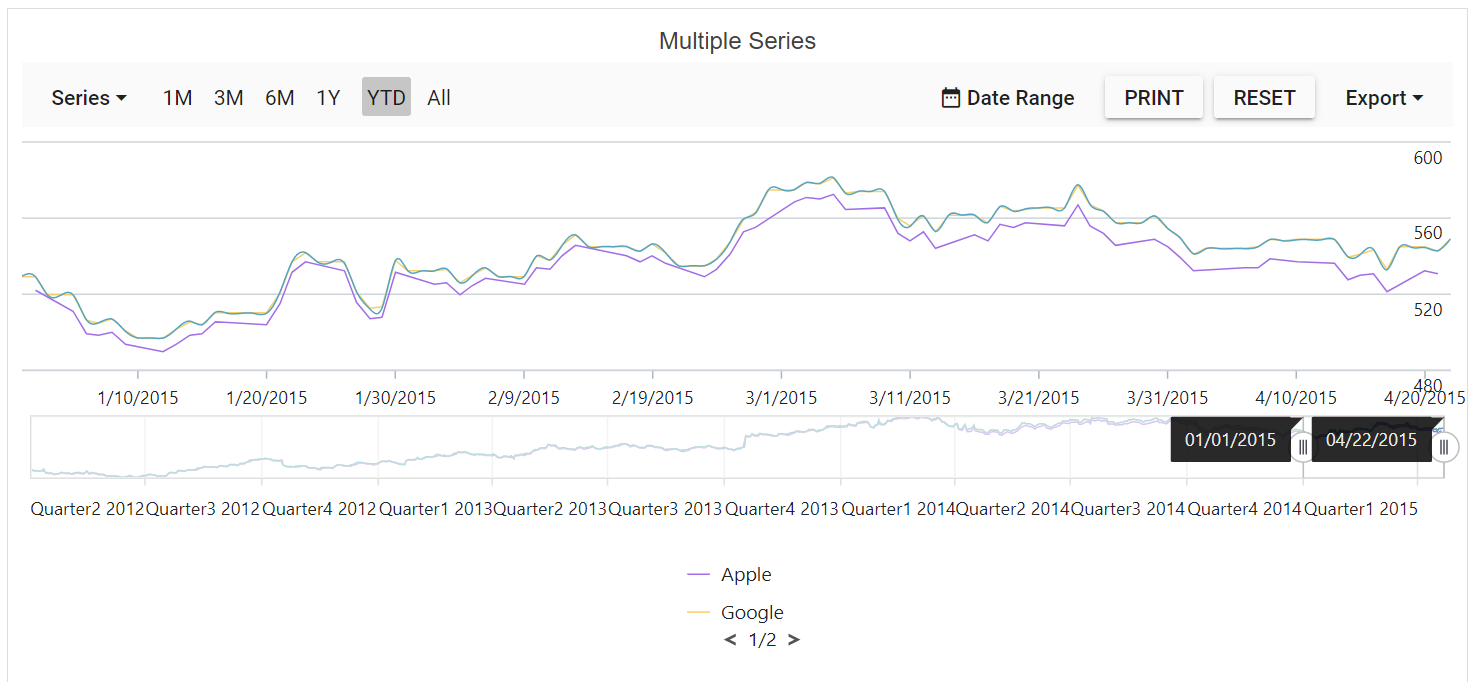### Legend Text Wrap

When the legend text exceeds the container, the text can be wrapped by using TextWrap property. End user can also wrap the legend text based on the MaxLabelWidth property.

``````@using Syncfusion.Blazor.Charts

<SfStockChart Title="AAPL Stock Price">
<StockChartLegendSettings Visible="true" Position="@LegendPosition.Right" TextWrap="@TextWrap.Wrap" MaxLabelWidth="70">
</StockChartLegendSettings>
<StockChartSeriesCollection>
<StockChartSeries DataSource="@StockDetails" Type="ChartSeriesType.Line" XName="Date" High="High" Low="Low" Open="Open" Close="Close" Name="Apple Stock Price"></StockChartSeries>
<StockChartSeries DataSource="@StockDetails" Type="ChartSeriesType.Line" XName="Date" High="High" Low="Low" Open="Open" Close="Close" Name="Google Stock Price"></StockChartSeries>
<StockChartSeries DataSource="@StockDetails" Type="ChartSeriesType.Spline" XName="Date" High="High" Low="Low" Open="Open" Close="Close" Name="Tesla Stock Price"></StockChartSeries>
</StockChartSeriesCollection>
</SfStockChart>

@code {
public class ChartData
{
public DateTime Date { get; set; }
public Double Open { get; set; }
public Double Low { get; set; }
public Double Close { get; set; }
public Double High { get; set; }
public Double Volume { get; set; }
}

public List<ChartData> StockDetails = new List<ChartData>
{
new ChartData { Date = new DateTime(2012, 04, 02), Open= 85.9757, High = 90.6657,Low = 85.7685, Close = 90.5257,Volume = 660187068},
new ChartData { Date = new DateTime(2012, 04, 09), Open= 89.4471, High = 92,Low = 86.2157, Close = 86.4614,Volume = 912634864},
new ChartData { Date = new DateTime(2012, 04, 16), Open= 87.1514, High = 88.6071,Low = 81.4885, Close = 81.8543,Volume = 1221746066},
new ChartData { Date = new DateTime(2012, 04, 23), Open= 81.5157, High = 88.2857,Low = 79.2857, Close = 86.1428,Volume = 965935749},
new ChartData { Date = new DateTime(2012, 04, 30), Open= 85.4, High =  85.4857,Low = 80.7385, Close = 80.75,Volume = 615249365},
};
}``````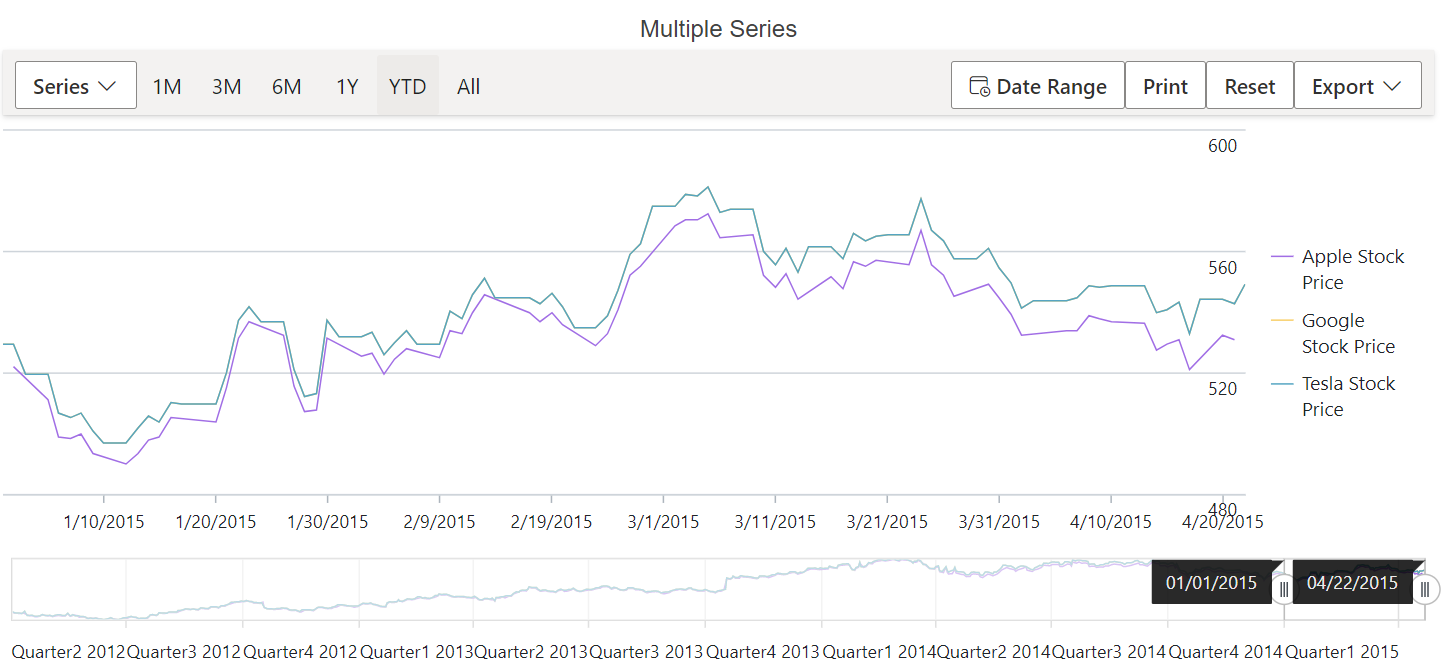## Series selection based on legend

By default, when you click on the legend item, the appropriate series visibility is collapsed. On the other hand, ToggleVisibility property is used to disable such functionality.

``````@using Syncfusion.Blazor.Charts

<SfStockChart Title="AAPL Stock Price" SelectionMode="SelectionMode.Series">
<StockChartLegendSettings Visible="true"  ToggleVisibility="false">
</StockChartLegendSettings>
<StockChartSeriesCollection>
<StockChartSeries DataSource="@StockDetails" Type="ChartSeriesType.Line" XName="Date" High="High" Low="Low" Open="Open" Close="Close" Name="Apple Stock Price"></StockChartSeries>
<StockChartSeries DataSource="@StockDetails" Type="ChartSeriesType.Line" XName="Date" High="High" Low="Low" Open="Open" Close="Close" Name="Google Stock Price"></StockChartSeries>
<StockChartSeries DataSource="@StockDetails" Type="ChartSeriesType.Spline" XName="Date" High="High" Low="Low" Open="Open" Close="Close" Name="Tesla Stock Price"></StockChartSeries>
</StockChartSeriesCollection>
</SfStockChart>

@code {
public class ChartData
{
public DateTime Date { get; set; }
public Double Open { get; set; }
public Double Low { get; set; }
public Double Close { get; set; }
public Double High { get; set; }
public Double Volume { get; set; }
}

public List<ChartData> StockDetails = new List<ChartData>
{
new ChartData { Date = new DateTime(2012, 04, 02), Open= 85.9757, High = 90.6657,Low = 85.7685, Close = 90.5257,Volume = 660187068},
new ChartData { Date = new DateTime(2012, 04, 09), Open= 89.4471, High = 92,Low = 86.2157, Close = 86.4614,Volume = 912634864},
new ChartData { Date = new DateTime(2012, 04, 16), Open= 87.1514, High = 88.6071,Low = 81.4885, Close = 81.8543,Volume = 1221746066},
new ChartData { Date = new DateTime(2012, 04, 23), Open= 81.5157, High = 88.2857,Low = 79.2857, Close = 86.1428,Volume = 965935749},
new ChartData { Date = new DateTime(2012, 04, 30), Open= 85.4, High =  85.4857,Low = 80.7385, Close = 80.75,Volume = 615249365},
};
}``````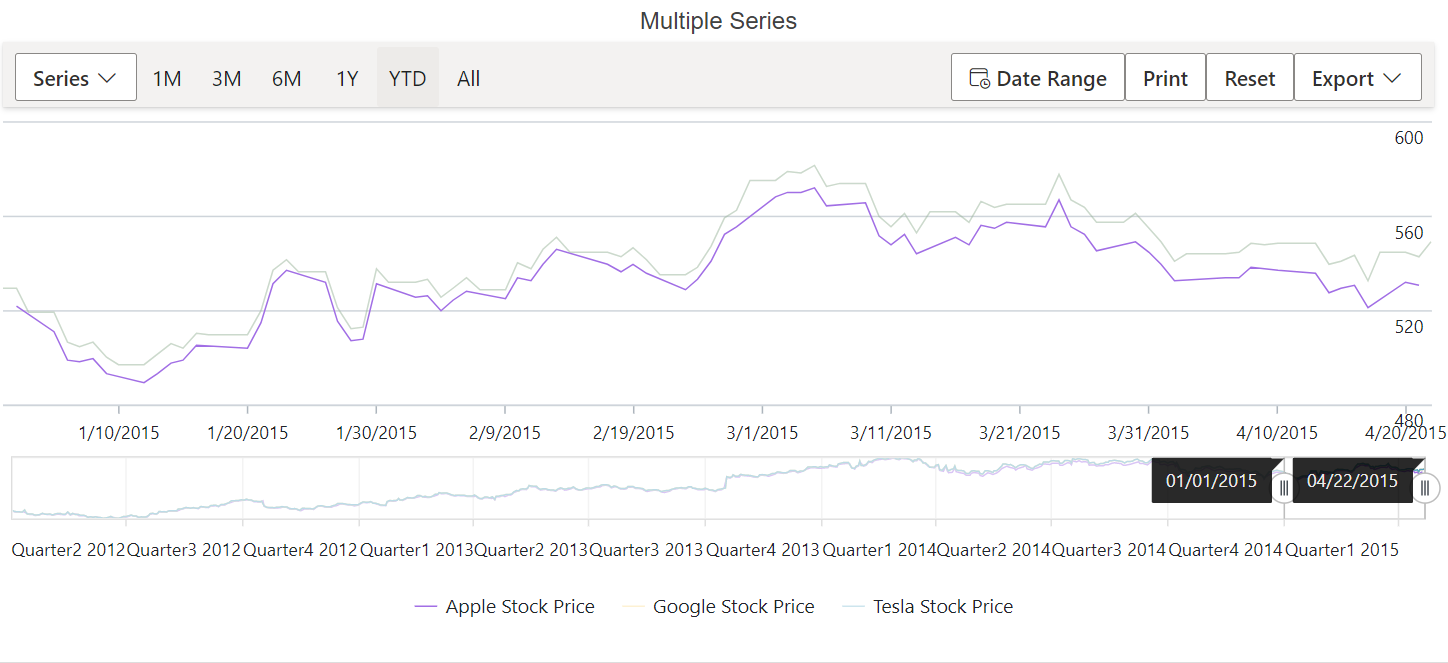## Hiding legend item

The series Name will be displayed as the legend text by default. You can skip the legend for particular series by providing an empty string to the series Name property.

``````@using Syncfusion.Blazor.Charts

<SfStockChart Title="AAPL Stock Price" SelectionMode="SelectionMode.Series">
<StockChartLegendSettings Visible="true"  ToggleVisibility="true">
</StockChartLegendSettings>
<StockChartSeriesCollection>
<StockChartSeries DataSource="@StockDetails" Type="ChartSeriesType.Line" XName="Date" High="High" Low="Low" Open="Open" Close="Close" Name="Apple Stock Price"></StockChartSeries>
<StockChartSeries DataSource="@StockDetails" Type="ChartSeriesType.Line" XName="Date" High="High" Low="Low" Open="Open" Close="Close" Name="Google Stock Price"></StockChartSeries>
<StockChartSeries DataSource="@StockDetails" Type="ChartSeriesType.Spline" XName="Date" High="High" Low="Low" Open="Open" Close="Close" Name="Tesla Stock Price"></StockChartSeries>
</StockChartSeriesCollection>
</SfStockChart>

@code {
public class ChartData
{
public DateTime Date { get; set; }
public Double Open { get; set; }
public Double Low { get; set; }
public Double Close { get; set; }
public Double High { get; set; }
public Double Volume { get; set; }
}

public List<ChartData> StockDetails = new List<ChartData>
{
new ChartData { Date = new DateTime(2012, 04, 02), Open= 85.9757, High = 90.6657,Low = 85.7685, Close = 90.5257,Volume = 660187068},
new ChartData { Date = new DateTime(2012, 04, 09), Open= 89.4471, High = 92,Low = 86.2157, Close = 86.4614,Volume = 912634864},
new ChartData { Date = new DateTime(2012, 04, 16), Open= 87.1514, High = 88.6071,Low = 81.4885, Close = 81.8543,Volume = 1221746066},
new ChartData { Date = new DateTime(2012, 04, 23), Open= 81.5157, High = 88.2857,Low = 79.2857, Close = 86.1428,Volume = 965935749},
new ChartData { Date = new DateTime(2012, 04, 30), Open= 85.4, High =  85.4857,Low = 80.7385, Close = 80.75,Volume = 615249365},
};
}``````